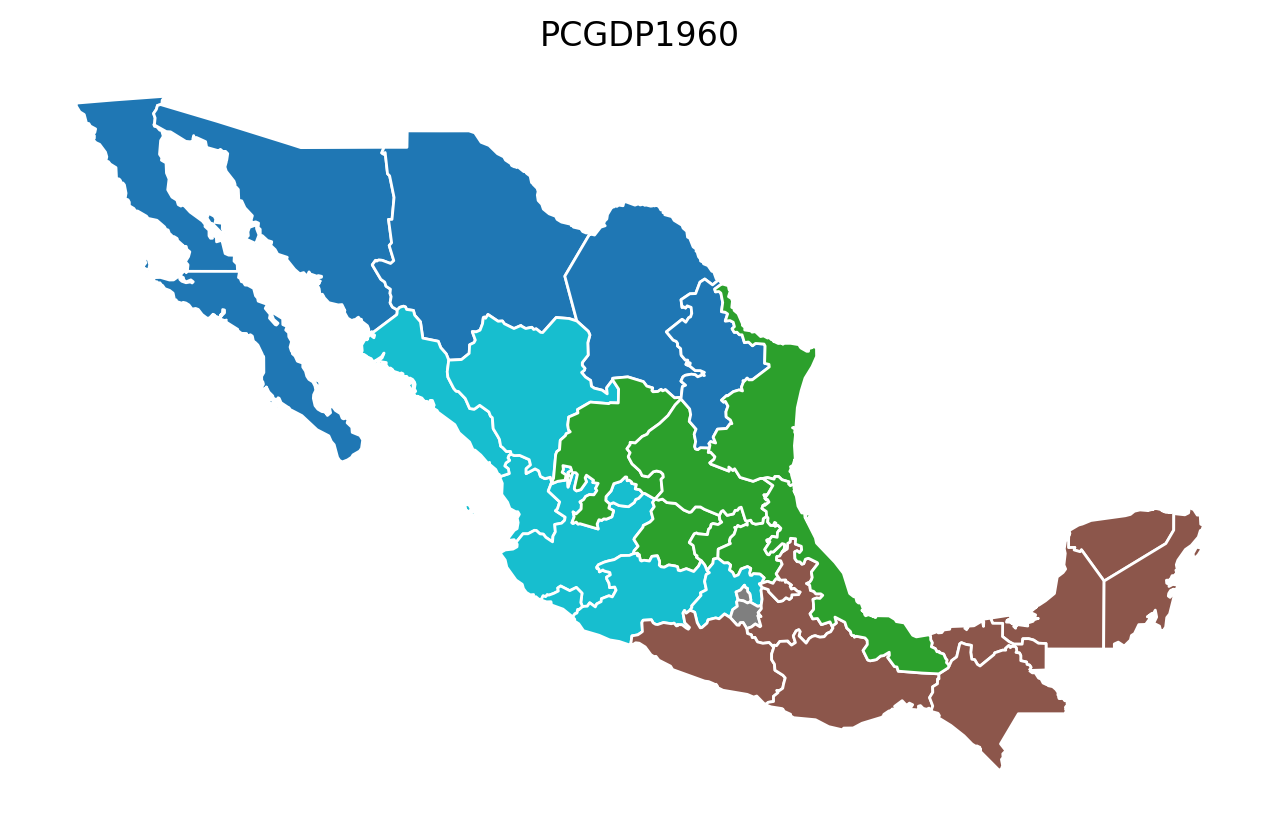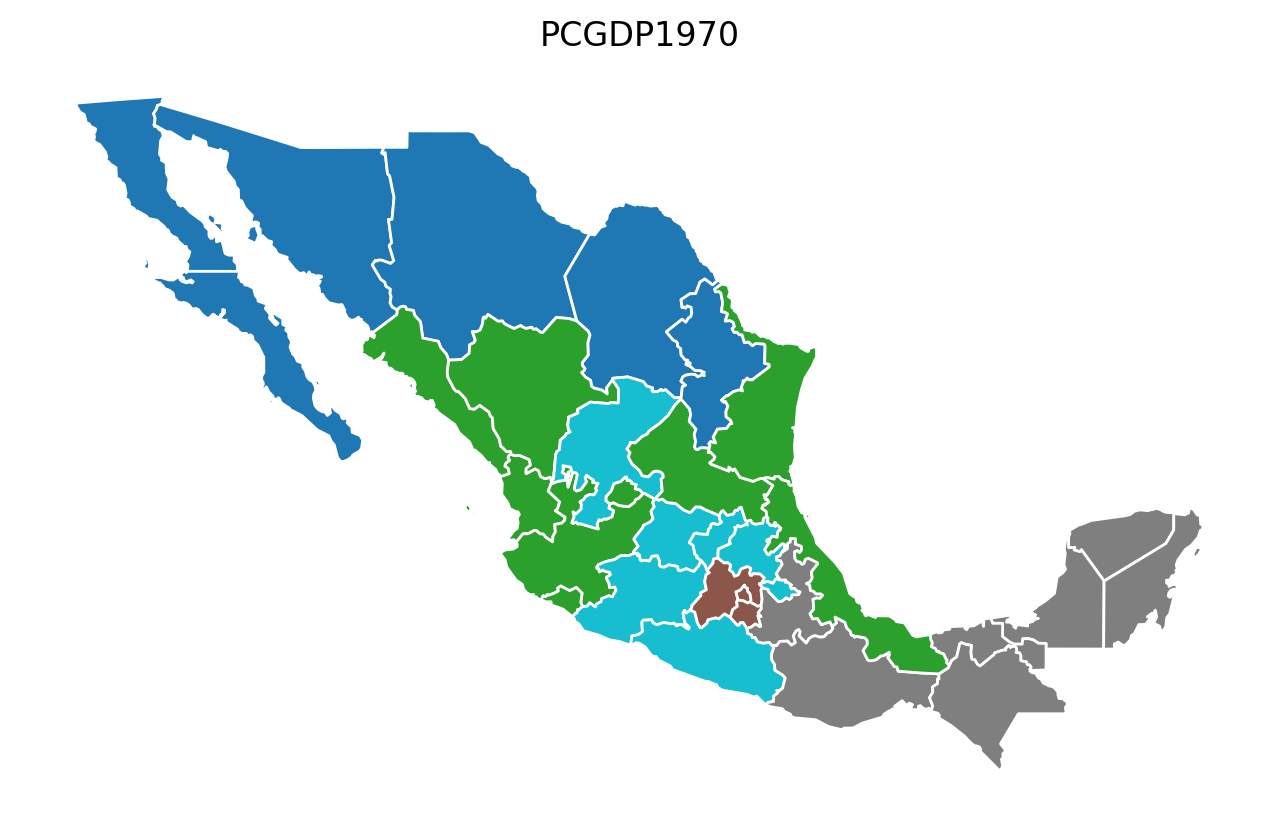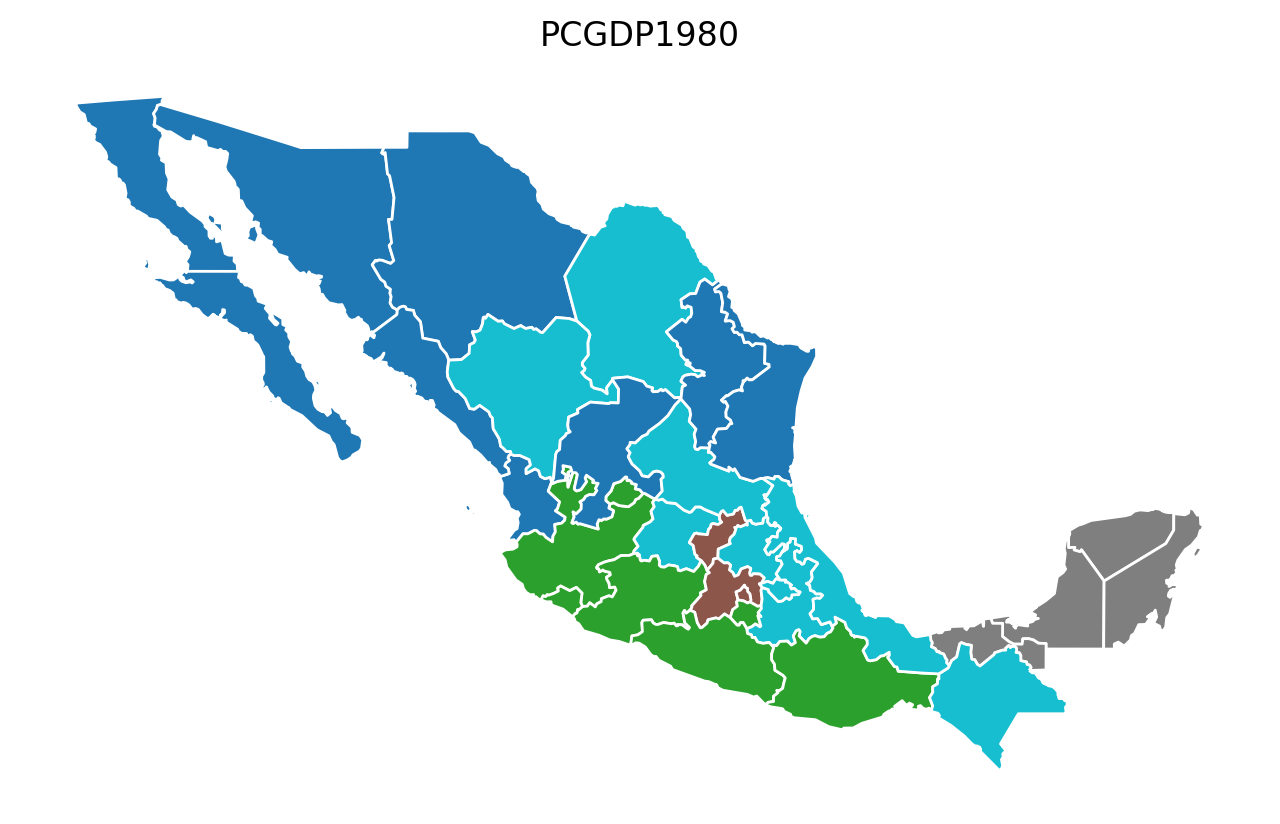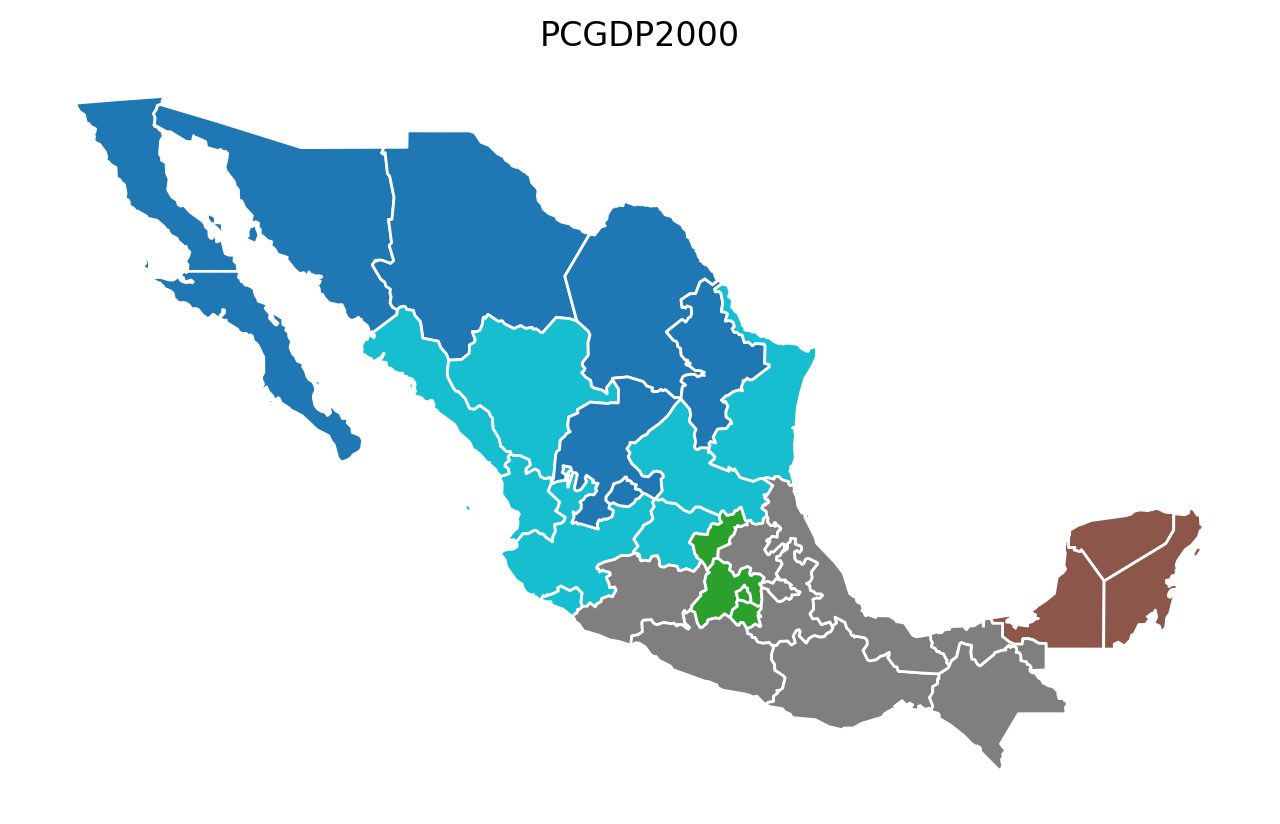This page was generated from notebooks/azp.ipynb. Interactive online version:# Automatic Zoning Procedure (AZP) algorithm¶

Authors:Xin Feng

AZP can work with different types of objective functions, which are very sensitive to aggregating data from a large number of zones into a pre-designated smaller number of regions.

AZP was originally formulated in Openshaw, 1977 and then extended in Openshaw, S. and Rao, L. (1995).

:

import warnings
warnings.filterwarnings('ignore')
import geopandas as gpd
import libpysal
import numpy as np

import sys
sys.path.append("../")
from spopt.region import AZP

:

import matplotlib.pyplot as plt
plt.rcParams['figure.figsize'] = [12, 8]


## Mexican State Regional Income Clustering¶

To illustrate azp we utilize data on regional incomes for Mexican states over the period 1940-2000, originally used in Rey and Sastré-Gutiérrez (2010).

We can first explore the data by plotting the per capital gross regional domestic product (in constant USD 2000 dollars) for each year in the sample, using a quintile classification:

:

pth = libpysal.examples.get_path('mexicojoin.shp')

:

for year in range(1940, 2010, 10):
ax = mexico.plot(column=f'PCGDP{year}', scheme='Quantiles', cmap='GnBu', edgecolor='b', legend=True)
_ = ax.axis('off')
plt.title(str(year))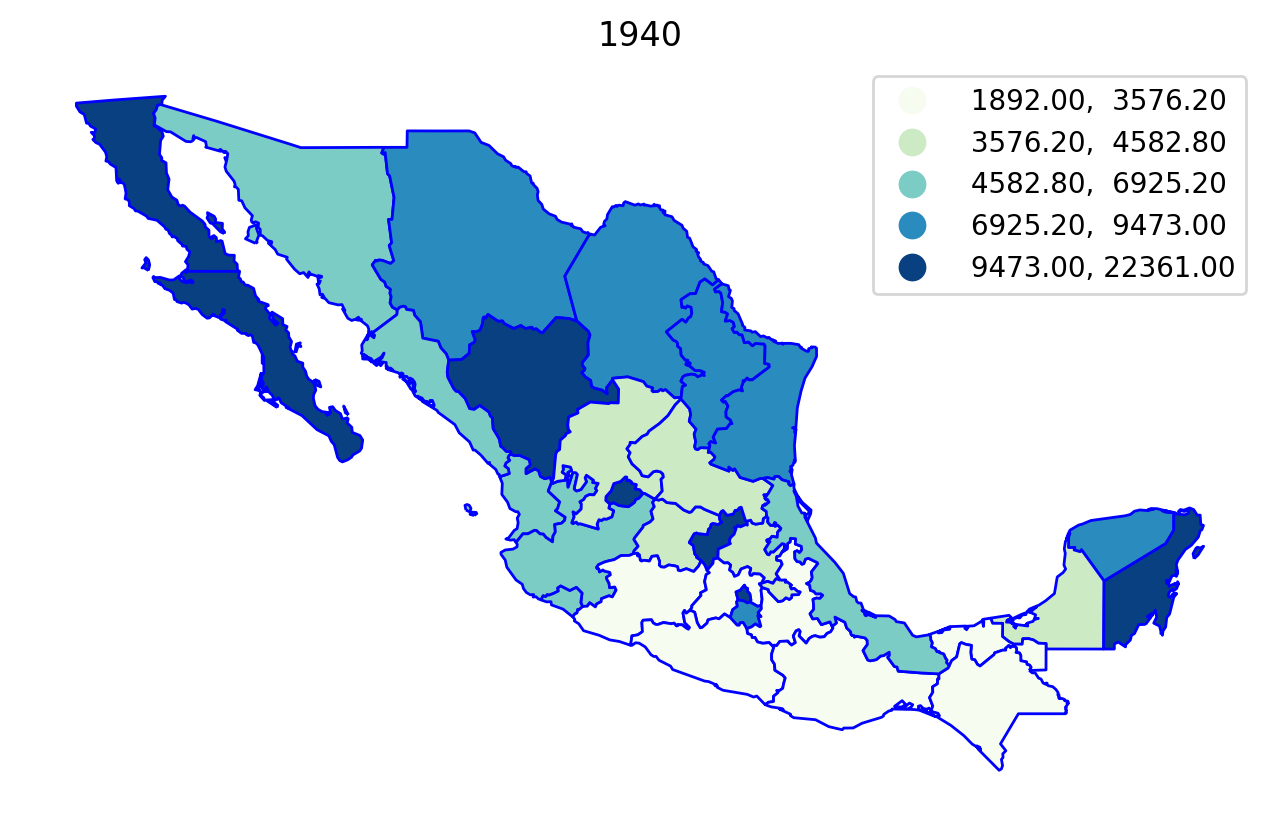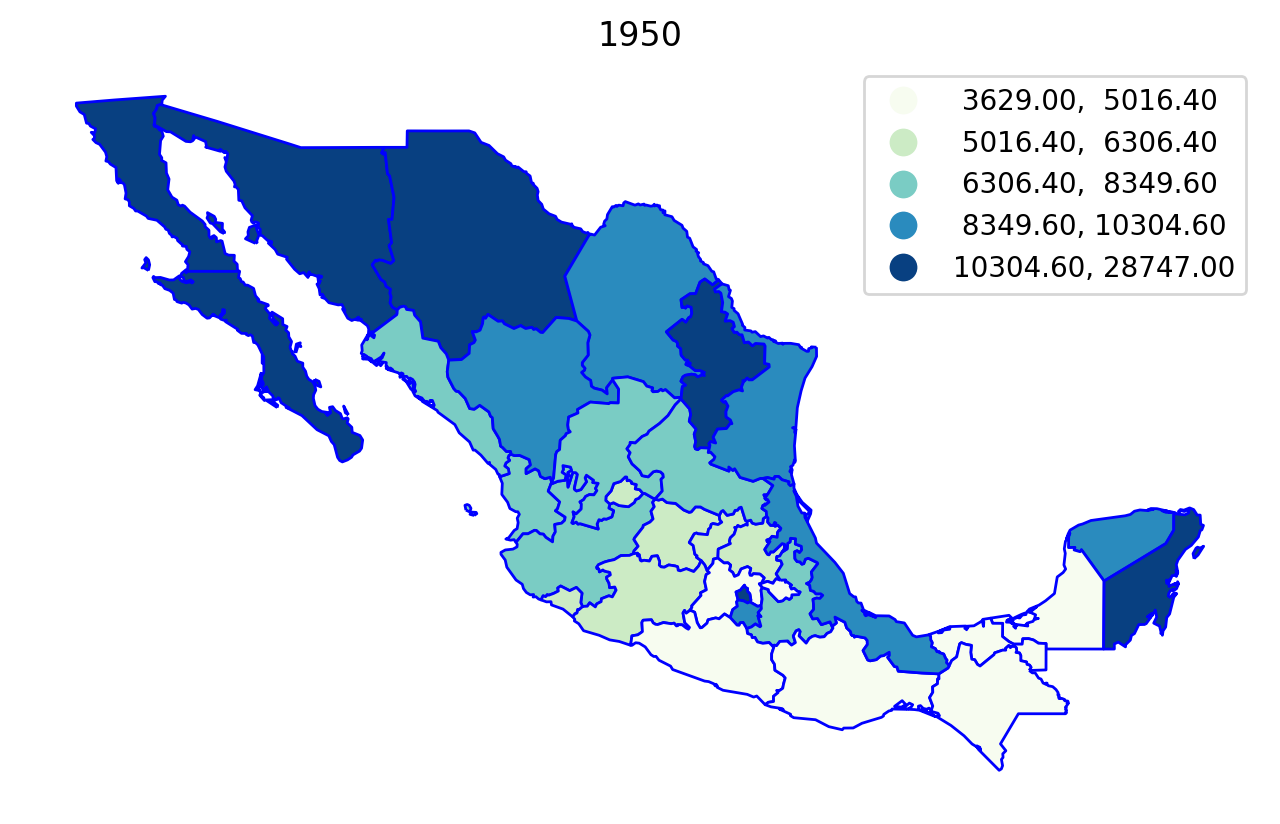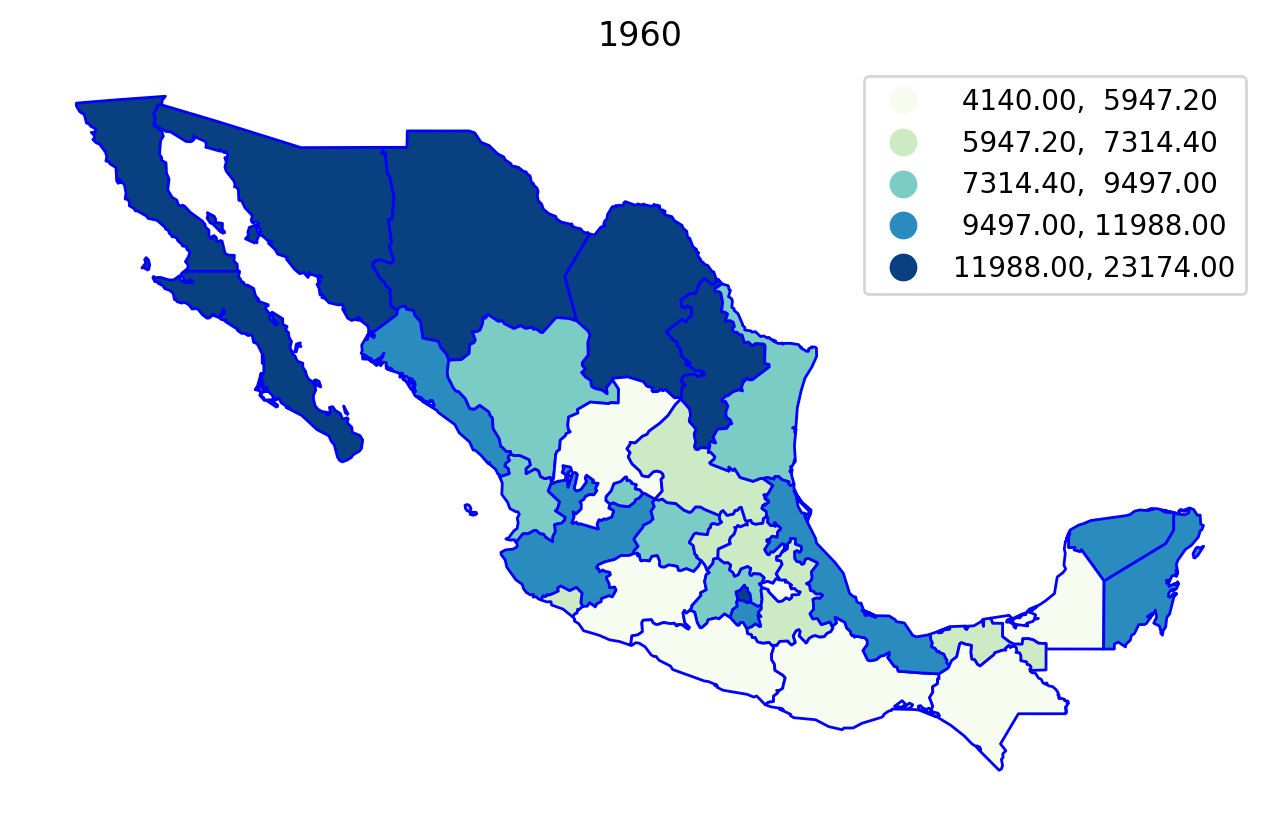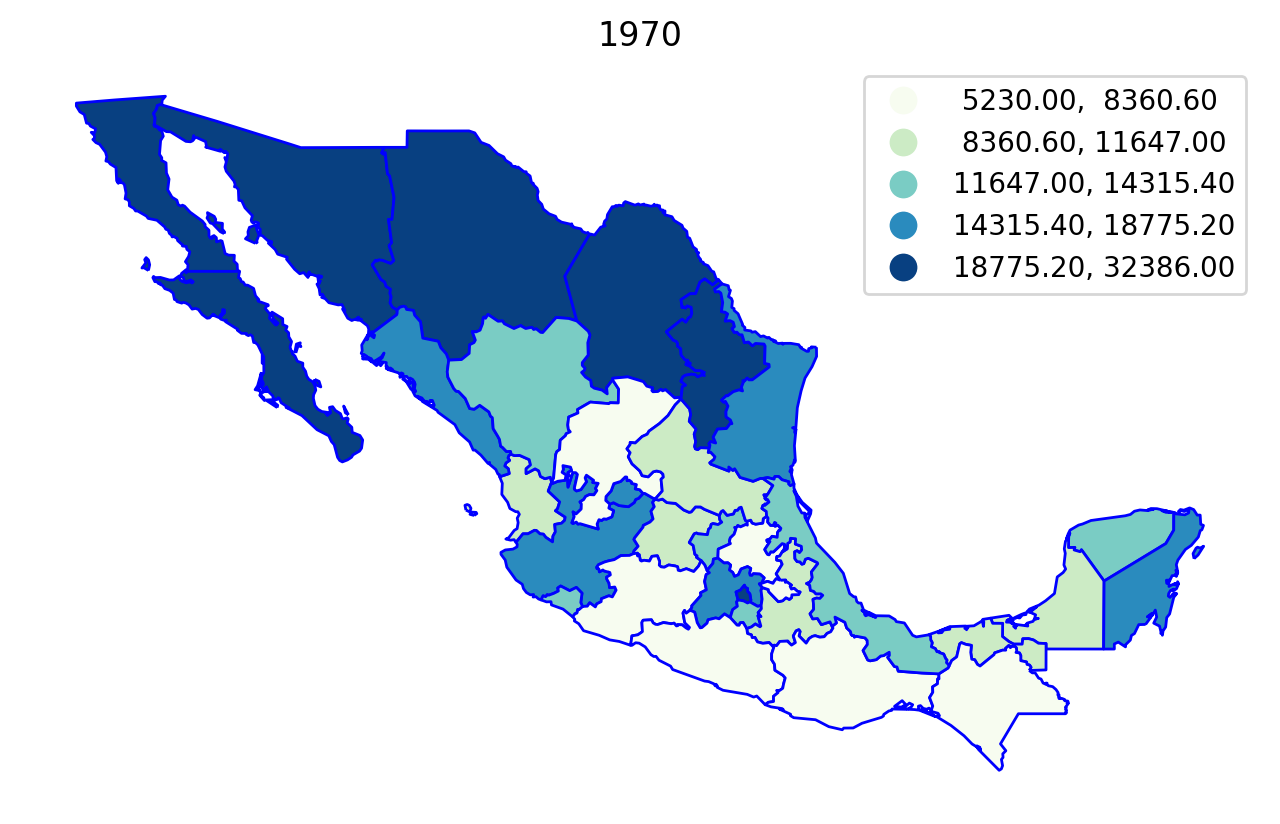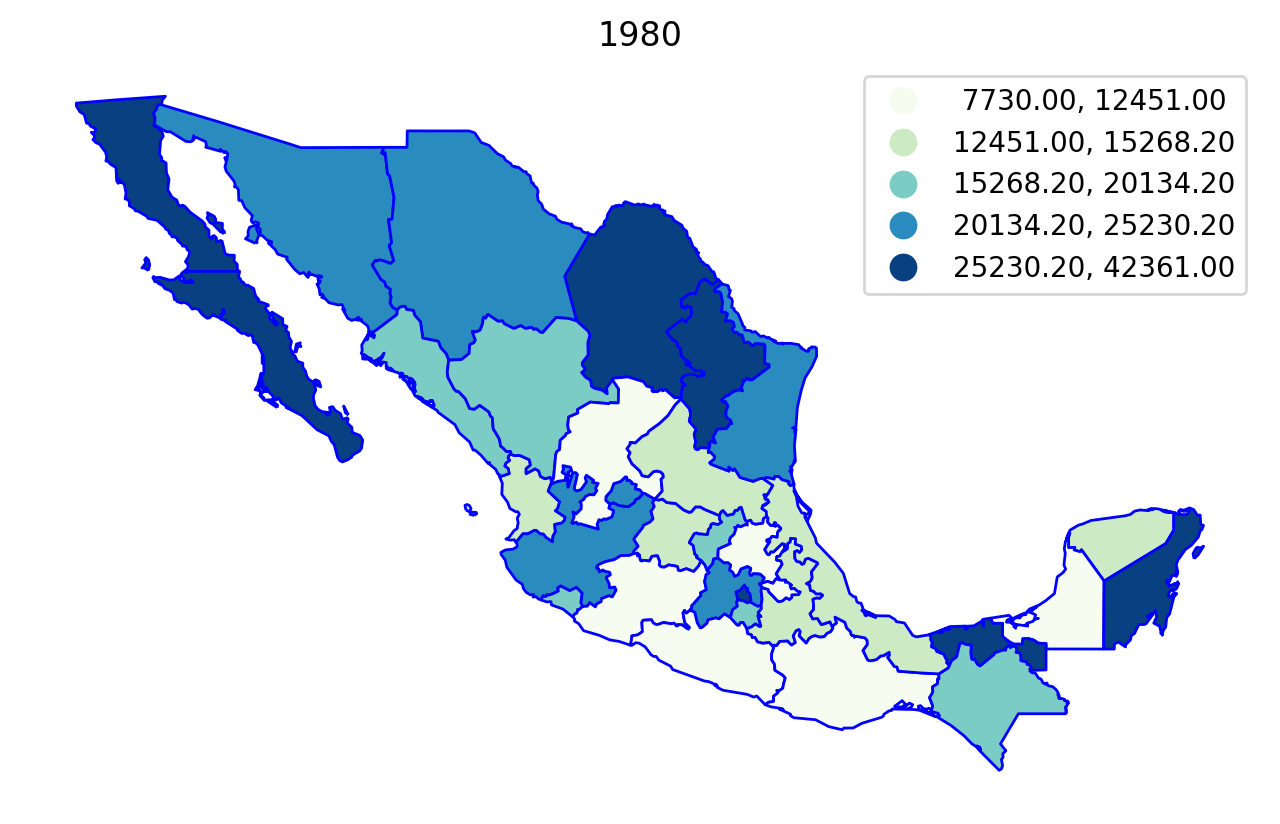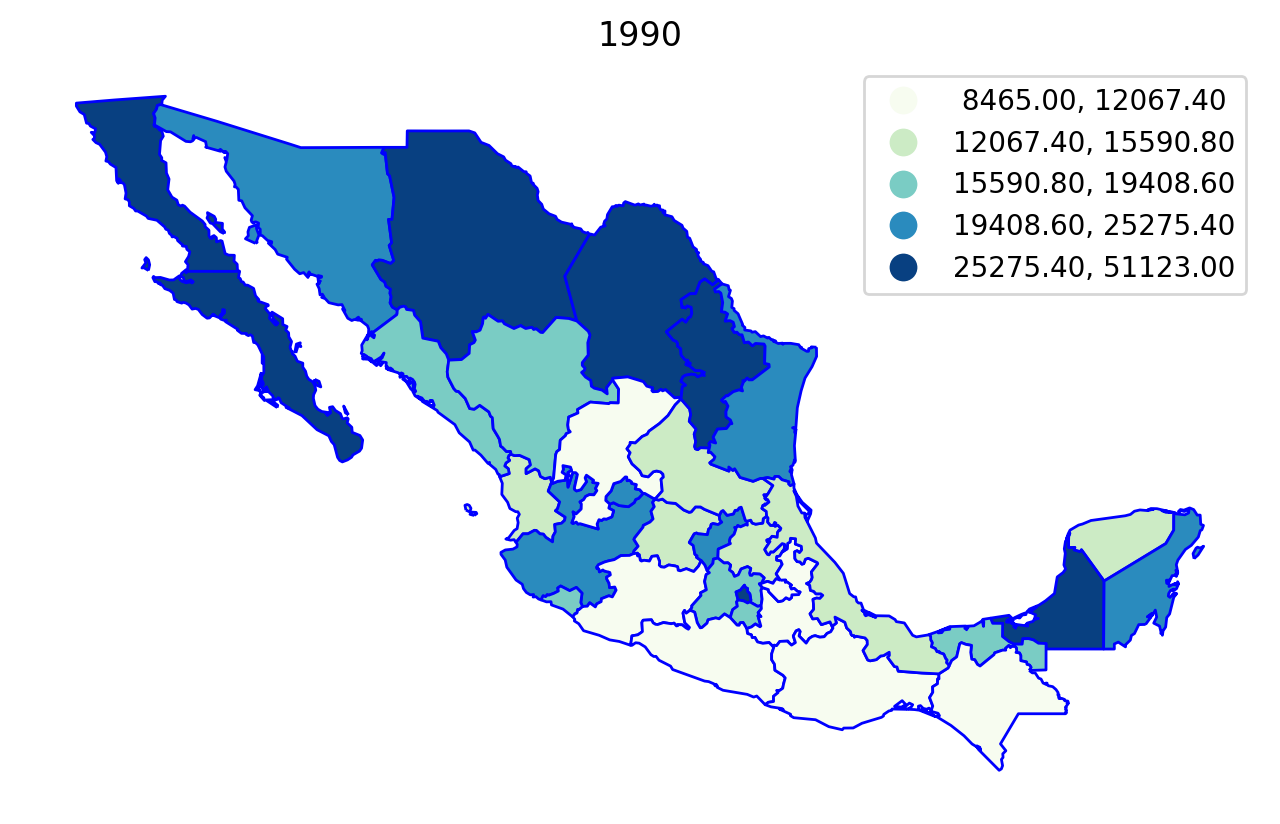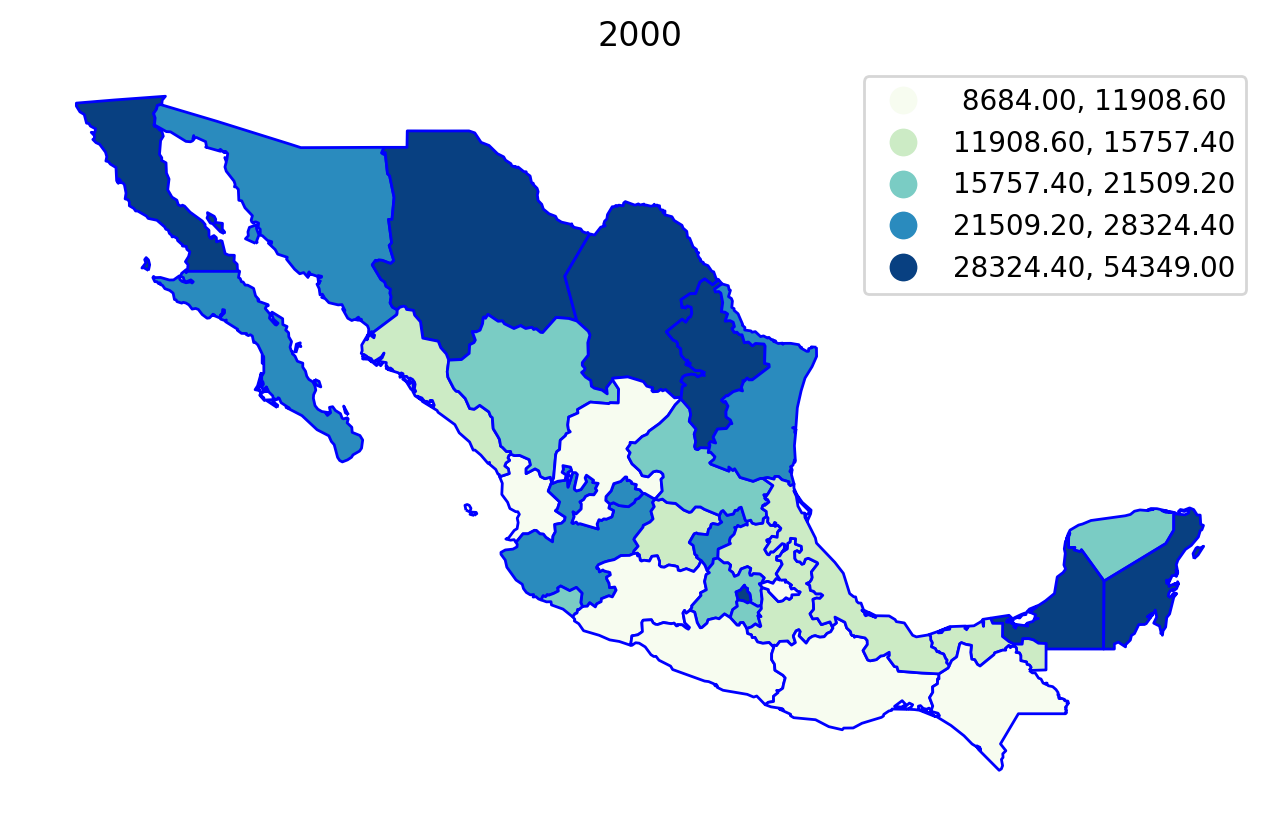## Regionalization¶

First, we specify a number of parameters that will serve as input to the azp model.

The variables in the dataframe that will be used to measure regional dissimilarity:

:

attrs_name = [f'PCGDP{year}' for year in range(1950,2010, 10)]
attrs_name

:

['PCGDP1950', 'PCGDP1960', 'PCGDP1970', 'PCGDP1980', 'PCGDP1990', 'PCGDP2000']


A spatial weights object expresses the spatial connectivity of the zones:

:

w = libpysal.weights.Queen.from_dataframe(mexico)


The number of regions that we would like to aggregate these zones into:

:

n_clusters = 5


There are four optional parameters. In this example, we only use the default settings, you can define them as needed.

allow_move_strategy: For a different behavior for allowing moves, an AllowMoveStrategy instance can be passed as argument.

class: AllowMoveStrategy or None, default: None


random_state: Random seed.

None, int, str, bytes, or bytearray, default: None


initial_labels: One-dimensional array of labels at the beginning of the algorithm.

class: numpy.ndarray or None, default: None
If None, then a random initial clustering will be generated.


objective_func: the objective function to use.

class: spopt.region.objective_function.ObjectiveFunction, default: ObjectiveFunctionPairwise()


The model can then be solved:

:

model = AZP(mexico, w, attrs_name, n_clusters)
model.solve()

n_regions_per_comp {0: 5}
comp_label 0
n_regions_in_comp 5
Regions in comp: {0, 1, 2, 3, 4}

:

mexico['azp_new'] = model.labels_

:

mexico['number'] = 1
mexico[['azp_new','number']].groupby(by='azp_new').count()

:

number
azp_new
0.0 5
1.0 8
2.0 9
3.0 5
4.0 5
:

mexico.plot(column='azp_new', categorical=True, edgecolor='w')

:

<AxesSubplot:>The model solution results in five regions, two of which have five states, one with four, one with eight, and one with ten states.

## Year-by-Year Regionalization (n_clusters = 5 regions)¶

:

for year in attrs_name:

model = AZP(mexico, w, year, 5)
model.solve()
lab = year+'labels_'
mexico[lab] = model.labels_
ax = mexico.plot(column=lab, categorical=True, edgecolor='w')
plt.title(year)
_ = ax.axis('off')

n_regions_per_comp {0: 5}
comp_label 0
n_regions_in_comp 5
Regions in comp: {0, 1, 2, 3, 4}
n_regions_per_comp {0: 5}
comp_label 0
n_regions_in_comp 5
Regions in comp: {0, 1, 2, 3, 4}
n_regions_per_comp {0: 5}
comp_label 0
n_regions_in_comp 5
Regions in comp: {0, 1, 2, 3, 4}
n_regions_per_comp {0: 5}
comp_label 0
n_regions_in_comp 5
Regions in comp: {0, 1, 2, 3, 4}
n_regions_per_comp {0: 5}
comp_label 0
n_regions_in_comp 5
Regions in comp: {0, 1, 2, 3, 4}
n_regions_per_comp {0: 5}
comp_label 0
n_regions_in_comp 5
Regions in comp: {0, 1, 2, 3, 4}